QUESTION: Is it my imagination, or does the IDLanROI object calculate a polygon mask incorrectly? Depending on the mask "rule," the polygon is either one pixel too small on a side, or one pixel too large on a side. It seems impossible to get a polygon mask that is "just right."ANSWER: You are right. I believe the mask is calculated incorrectly. To see what I mean, let's try calculating a polygon and creating a "mask" with direct graphics routines first. Here is a polygon that goes from 5 to 10 in both X and Y directions.

poly = [[5, 10, 10, 5, 5], [5, 5, 10, 10, 5]]

Here is code that displays the polygon as a filled polygon.

cgDisplay, 400, 400, WID=0, Title = 'Polygon Fill'
cgPlot, , XRange=[0,20], YRange=[0,20], /NoData, ASPECT=1.0, \$
YTickLen=1.0, XTickLen=1.0
cgPolygon, poly[*,0], poly[*,1], COLOR='steel blue', /Fill

You see the result in the figure below.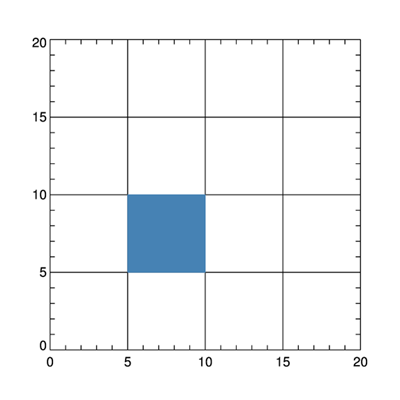A filled polygon. This is what we expect.

Now, for sanity's sake, let's try to create a pixel "mask" with PolyFillV. Here is the code.

cgDisplay, 400, 400, WID=1, Title='Polygon Pixel Fill - PolyFillV'
testImage = BytArr(20,20)+1B
pixels = PolyfillV(poly[*,0], poly[*,1], 20, 20)
testImage[pixels]=255
TVLCT, cgColor('red6', /Triple), 255
TVLCT, cgColor('white', /Triple), 1
cgPlot, , XRange=[0,20], YRange=[0,20], /NoData, ASPECT=1.0
cgImage, testImage, XRange=[0,20], YRange=[0,20], /Overplot
cgPlot, , XRange=[0,20], YRange=[0,20], /NoData, ASPECT=1.0, \$
YTickLen=1.0, XTickLen=1.0, /NoErase

You see the results in the figure below. It is identical to the first method.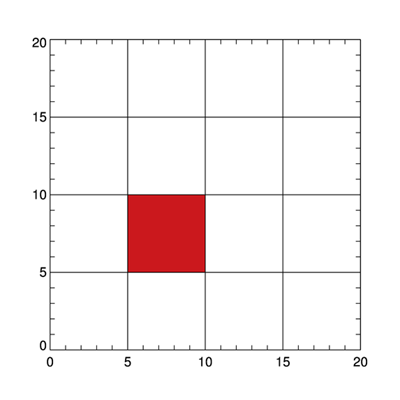A filled pixel mask created with PolyFillV. Also expected.

Now, let's use the same polygon and try to create a pixel mask with IDLanROI. First, let's try to use polygon internal pixels only by setting the MASK_RULE keyword to 1. Here is the code.

cgDisplay, 400, 400, WID=2, Title='Polygon Interior Pixel Fill - IDLanROI'
p = OBJ_NEW('IDLanROI', poly[*,0], poly[*,1])
anImage = BytArr(20,20)+1B
anImage[pixels] = 255
cgPlot, , XRange=[0,20], YRange=[0,20], /NoData, ASPECT=1.0
cgImage, anImage, XRange=[0,20], YRange=[0,20], /Overplot
cgPlot, , XRange=[0,20], YRange=[0,20], /NoData, ASPECT=1.0, \$
YTickLen=1.0, XTickLen=1.0, /NoErase

You see the results in the figure below. You can see a row of pixels is missing on the left and bottom sides of the polygon.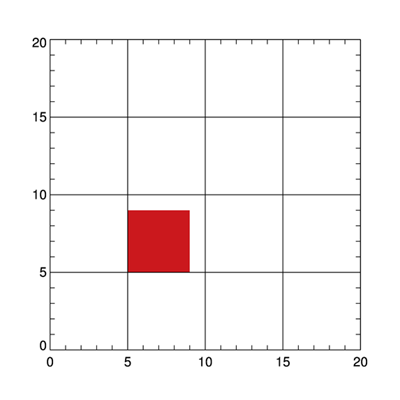An IDLanROI pixel mask with internal pixels. The polygon is too small.

If we change the pixel center (by, for example, setting the PIXEL_CENTER keyword to [1.0,1.0]), we move the location of the pixel mask, but we don't change its incorrect shape.

Suppose we try to make a mask from both the internal pixels and the boundary pixels. We do this by changing the MASK_RULE keyword to 2. Here is the code.

cgDisplay, 400, 400, WID=3, Title='Polygon All Pixel Fill - IDLanROI'
p = OBJ_NEW('IDLanROI', poly[*,0], poly[*,1])
anImage = BytArr(20,20)+1B
anImage[pixels] = 255
cgPlot, , XRange=[0,20], YRange=[0,20], /NoData, ASPECT=1.0
cgImage, anImage, XRange=[0,20], YRange=[0,20], /Overplot
cgPlot, , XRange=[0,20], YRange=[0,20], /NoData, ASPECT=1.0, \$
YTickLen=1.0, XTickLen=1.0, /NoErase

You see the results in the figure below. Now the polygon is too big by another row of pixels on the top and right sides.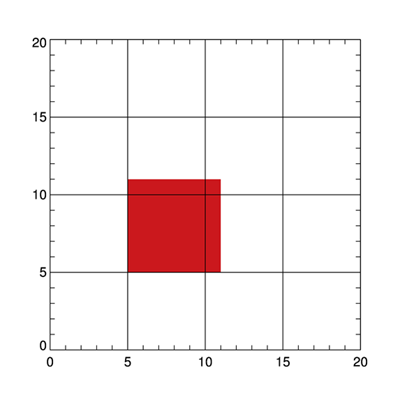An IDLanROI pixel mask with internal and boundary pixels. The polygon is too big.

It appears to be impossible to get a correct polygon mask from the IDLanROI object without somehow modifying the polygon values themselves.An Alternative Point of View

My friend, Fabien, offers an alternative point of view. He suggests that the behavior of IDLanROI is internally consistent. He offers as evidence, the ROIPolygonAlternativeView program as an example. He argues that the problem is not with IDLanROI, but with how the mask is drawn. He notes:

The IDLanROI object subscribes to a PIXEL CENTER view. So a pixel is touched from -0.5 to +0.5. IDL's graphics pixels are following a lower-left pixel convention. PIXEL_CENTER does not change anything to this fact, it just shifts the whole grid. This means that the three mask rules are perfectly coherent with each other.
Running his program gives these results, with MASK_RULE going from 0 to 2 in a left to right direction (i.e, boundary pixels, internal pixels, and both boundary and internal pixels, with the actual polygon drawn in green in the figure).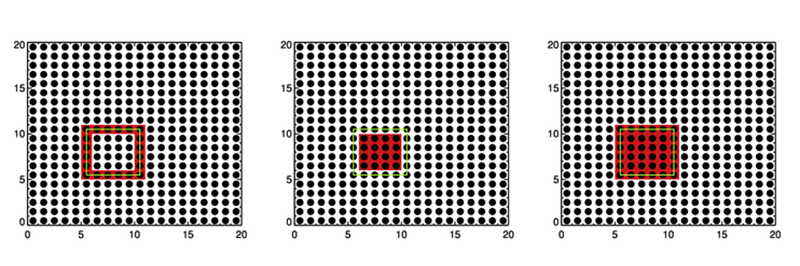A consistent story?

I'm prepared to admit the story is consistent. I would argue, however, that the result is so non-intuitive given all the ways we interact with polygons in the real world, that the result is a de facto bug.

Even Function Graphics gets the notion of a polygon right, a result that seals the deal for me in calling this a bug in the IDLanROI code.

poly = [[5, 10, 10, 5, 5], [5, 5, 10, 10, 5]]
aplot = Plot(, XRange=[0,20], YRange=[0,20], /NoData, \$
YTickLen=1.0, XTickLen=1.0)
apoly = Polygon(poly[*,0], poly[*,1], /FILL_BACKGROUND, \$
FILL_COLOR='Steel Blue', /DATA)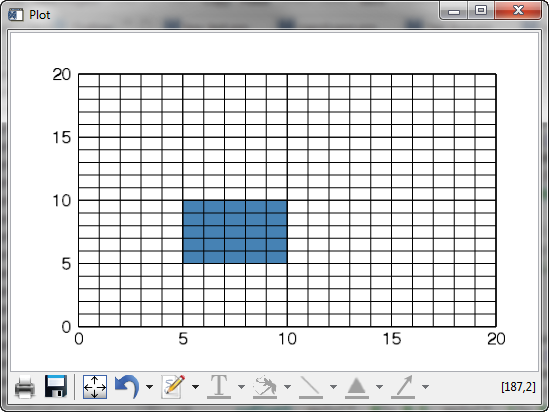Even function graphics gets it right.Further Explanation

Fabien provided another program that gives, he thinks, a better explanation of how the IDLanROI object works. You see the results of the program in the figure below.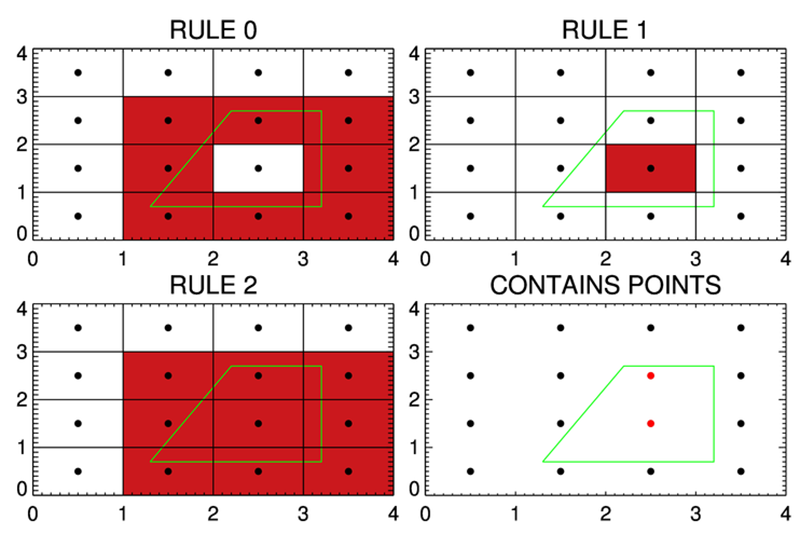Another view of how IDLanROI works.

All I really wish is that the IDLanROI object with a MASK_RULE=1 would return the same pixel identifiers as PolyFillV. That would make life a whole lot easier for me to understand!